#### How to Apply a Formula to an Entire Column in Excel

In this article, you will learn how to copy a formula into every cell in a column or row.

## Drag and Drop

Copying values in tables is easy. Take a look at the picture below. You should only put the cursor at the right bottom of the cell and double click.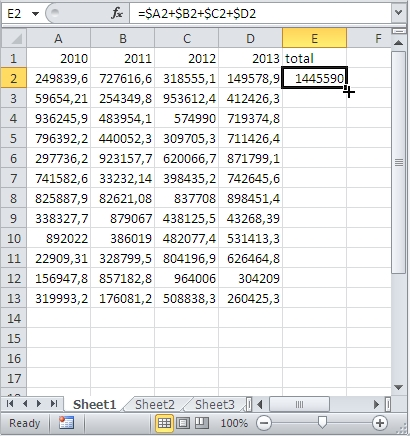Values are inserted only in the cells that have neighboring cells with data.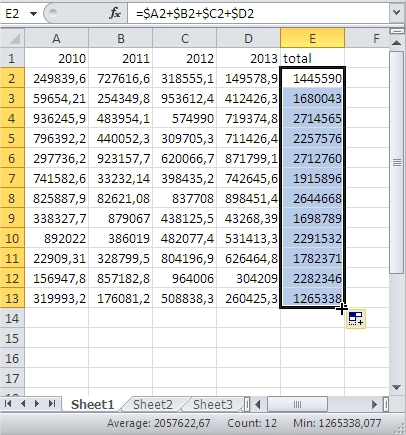## Double-click

Another option is more convenient. This is a good choice for large data sets when drag and drop way is not an option.

Start by positioning the cursor over the bottom-right corner of the cell. Your cursor should look like in the picture below.

The difference is that instead of dragging you need to double-click this corner.Excel applied the formula to the entire column. It is alligning to the column in the left hand side. You can see that Column D contains the data until the row number thirteen. This is exactly where Excel will reach the same in the Column E.

## Fill down

But what to do when you want to copy an entire column or row? There is a Fill option in the Ribbon in Excel.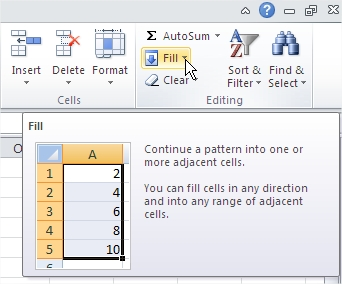Put your formula in the first row of the entire column. Select the whole column and use Fill > Down column.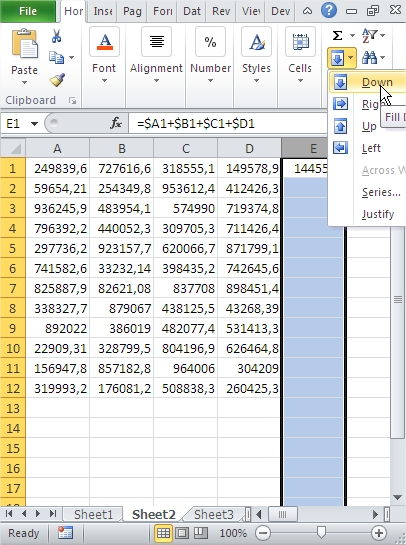As you see, you copied the formula into every cell in the column. Also, below are 12 rows where you don’t have any neighbors in column D.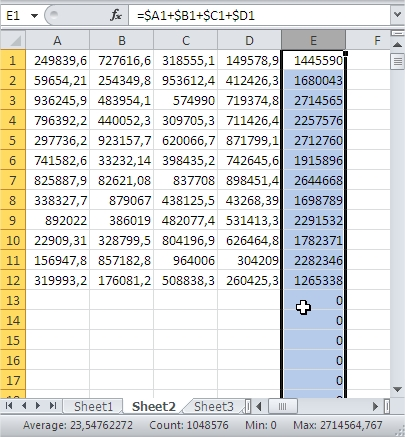You can also copy an entire row the same way. Use Fill > Right then instead Fill > Down.

It is important to note that the formula will be applied to all of the cells in the column until it reaches the last occupied cell. If you want to apply the formula to a specific number of cells, you can select those cells before dragging the Fill Handle.

## Using Excel Tables

Excel Tables, also known as ListObjects, are a powerful feature that simplifies working with data. They automatically expand to include new data and allow you to apply formulas efficiently. Here’s how to use them:

1. Select your dataset, including the column where you want to apply the formula.
2. Go to the “Insert” tab and choose “Table” or press Ctrl + T.

Now, you have a structured Excel Table, and any formula you apply in a column is automatically extended as new data is added below. Excel handles this expansion dynamically.

## Using Excel Tables with Structured References

Structured references are a feature of Excel Tables that allow you to refer to table columns in a more readable and dynamic way. You can use them in formulas to apply calculations across entire columns. Here’s how:

1. Assume you have a table named “SalesData” with columns “Product” and “Revenue.”
2. To calculate the total revenue for all products, enter the formula =SUM(SalesData[Revenue]) in a cell outside the table.

This formula uses structured references to refer to the “Revenue” column in the “SalesData” table. As new data is added to the table, the formula updates automatically.

## Using Defined Names

Another advanced method involves using Defined Names (also known as Named Ranges) to apply formulas to entire columns. Here’s how to set it up:

1. Select the entire column where you want to apply the formula.
2. In the “Formulas” tab, click “Define Name” or press Ctrl + F3.
3. Give the name a descriptive title, like “TotalRevenue.”
4. In the “Refers to” field, enter your formula. For example, =SUM(Sheet1!\$B\$2:INDEX(Sheet1!\$B:\$B,COUNTA(Sheet1!\$B:\$B))).

Now, you can use the defined name, “TotalRevenue,” in your formulas. It will automatically adjust to accommodate new data added to the column.

## Using Dynamic Array Formulas

You can leverage dynamic array formulas to apply calculations to entire columns without worrying about the exact number of rows. For example, to calculate the total revenue for all products in a column:

In a cell, enter the formula =SUM(SalesData[Revenue]).

Excel will automatically spill the result over multiple cells below the formula, covering all the relevant data in the column.

These methods ensure that your calculations remain accurate and dynamic, even as your data grows or changes. Choose the approach that best suits your specific needs to work more efficiently with your data.